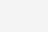# Python入门学习 | 深入 Python 字典并学习其内部实现

Python字典是Python入门学习过程中非常重要的一个知识点，今天给大家推荐的这篇文章就是Python字典的学习。深入到Python字典的内部实现，对于大家理解和学习Python是很有帮助的。

>>> d = {‘a’: 1, ‘b’: 2}
>>> d[‘c’] = 3
>>> d
{‘a’: 1, ‘b’: 2, ‘c’: 3}

>>> d[‘a’]
1
>>> d[‘b’]
2
>>> d[‘c’]
3
>>> d[‘d’]
Traceback (most recent call last):
File “<stdin>”, line 1, in <module>
KeyError: ‘d’

>>> map(hash, (0, 1, 2, 3))
[0, 1, 2, 3]
>>> map(hash, (“namea”, “nameb”, “namec”, “named”))
[1658398457, 1658398460, 1658398459, 1658398462]

arguments: string object
returns: hash
function string_hash:
if hash cached:
return it
set len to string’s length
initialize var p pointing to 1st char of string object
set x to value pointed by p left shifted by 7 bits
while len >= 0:
set var x to (1000003 * x) xor value pointed by p
increment pointer p
set x to x xor length of string object
cache x as the hash so we don’t need to calculate it again
return x as the hashj = (5*j) + 1 + perturb;
perturb >>= PERTURB_SHIFT;
use j % 2**i as the next table index;typedef struct {
Py_ssize_t me_hash;
PyObject *me_key;
PyObject *me_value
} PyDictEntry;

typedef struct _dictobject PyDictObject;
struct _dictobject {
Py_ssize_t ma_fill;
Py_ssize_t ma_used;
PyDictEntry *ma_table;
PyDictEntry *(*ma_lookup)(PyDictObject *mp, PyObject *key, long hash);
PyDictEntry ma_smalltable[PyDict_MINSIZE];
};

returns new dictionary object
function PyDict_New:
allocate new dictionary object
clear dictionary‘s table
set dictionary’s number of used slots + dummy slots (ma_fill) to 0
set dictionary‘s number of active slots (ma_used) to 0
set dictionary’s mask (ma_value) to dictionary size 1 = 7
set dictionarys lookup function to lookdict_string
return allocated dictionary object

arguments: dictionary, key, value
returns: 0 if OK or 1
function PyDict_SetItem:
if key‘s hash cached:
use hash
else:
calculate hash
call insertdict with dictionary object, key, hash and value
if key/value pair added successfully and capacity over 2/3:
call dictresize to resize dictionary’s table

inserdict() 使用搜寻函数 lookdict_string() 来查找空闲槽。这跟查找键所用的是同一函数。lookdict_string() 使用哈希值和掩码计算槽的索引。如果用“索引 = 哈希值&掩码”的方法未找到键，则会用调用先前介绍的循环方法探测，直至找到一个空闲槽。第一轮探测，如果未找到匹配的键的且探测过程中遇到过哑槽，则返回一个哑槽。这可使优先选择先前删除的槽。

• `PyDict_SetItem`: key = ‘a’, value = 1
• hash = hash(‘a’) = 12416037344
• insertdict
• lookdict_string
• slot index = hash & mask = 12416037344 & 7 = 0
• slot 0 is not used so return it
• init entry at index 0 with key, value and hash
• ma_used = 1, ma_fill = 1
• `PyDict_SetItem`: key = ‘b’, value = 2
• hash = hash(‘b’) = 12544037731
• insertdict
• lookdict_string
• slot index = hash & mask = 12544037731 & 7 = 3
• slot 3 is not used so return it
• init entry at index 3 with key, value and hash
• ma_used = 2, ma_fill = 2
• `PyDict_SetItem`: key = ‘z’, value = 26
• hash = hash(‘z’) = 15616046971
• insertdict
• lookdict_string
• slot index = hash & mask = 15616046971 & 7 = 3
• slot 3 is used so probe for a different slot: 5 is free
• init entry at index 5 with key, value and hash
• ma_used = 3, ma_fill = 3
• `PyDict_SetItem`: key = ‘y’, value = 25
• hash = hash(‘y’) = 15488046584
• insertdict
• lookdict_string
• slot index = hash & mask = 15488046584 & 7 = 0
• slot 0 is used so probe for a different slot: 1 is free
• init entry at index 1 with key, value and hash
• ma_used = 4, ma_fill = 4
• `PyDict_SetItem`: key = ‘c’, value = 3
• hash = hash(‘c’) = 12672038114
• insertdict
• lookdict_string
• slot index = hash & mask = 12672038114 & 7 = 2
• slot 2 is free so return it
• init entry at index 2 with key, value and hash
• ma_used = 5, ma_fill = 5
• `PyDict_SetItem`: key = ‘x’, value = 24
• hash = hash(‘x’) = 15360046201
• insertdict
• lookdict_string
• slot index = hash & mask = 15360046201 & 7 = 1
• slot 1 is used so probe for a different slot: 7 is free
• init entry at index 7 with key, value and hash
• ma_used = 6, ma_fill = 68个槽中的6个已被使用，使用量已经超过了总容量的2/3，因而，dictresize()函数将会被调用，用以分配一个长度更大的数组，同时将旧表中的条目复制到新的表中。